### Day 15 - Secant vs. Tangent Lines - 01.25.16

 Update New Seats!Remediation ProcedureClass RadioUnit 1 TestAverage = 78% What is a secant line?the instantaneous rate of changethe average rate of changethe slope of a linethe equation of a linestraight line joining two points of a graphed functionWhat is a tangent line?the instantaneous rate of changethe average rate of changethe slope of a linethe equation of a linestraight line based on the slope at one pointFind the slope of the line based on these two points: (0,1) and (3,-4).5/33/5-3/5-5/3none of the aboveFind the equation of the line based on these two points: (0,1) and (3,-4).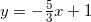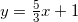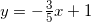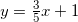none of the aboveIf the slope of a line is 4 and a point on the line is (4,-3), what is the equation of the line?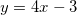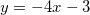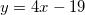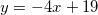none of the aboveReviewPre-calculusSlopeEquation of a LineSecant Line vs. Tangent Line (video)LessonChallenge 5Secant Line vs. Tangent Line ActivityEquation of a Tangent Line (video)Tangent Line GraphDerivative (video)Checkpoints N/AHomeworkStudy! Help Request List Standard(s) APC.2Define and apply the properties of limits of functions.Limits will be evaluated graphically and algebraically.Includes:​limits of a constant​limits of a sum, product, and quotient​one-sided limits​limits at infinity, infinite limits, and non-existent limitsAPC.3Use limits to define continuity and determine where a function is continuous or discontinuous.Includes:​continuity in terms of limitscontinuity at a point and over a closed interval​application of the Intermediate Value Theorem and the Extreme Value Theorem​geometric understanding and interpretation of continuity and discontinuity